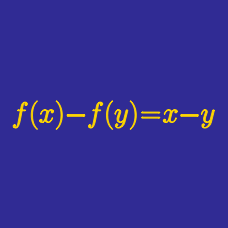Algebra

# Functional Equations - Introduction

Define the function $f^1(x) = \frac{1}{1-x}$, and $f^n (x) = f^1 ( f^{n-1} (x) )$ for positive integers $n$.

Evaluate $f^{36} ( 10 ) .$

Find all functions $f: \mathbb{R} \rightarrow \mathbb{R}$ such that

$5 f( x + y) + y^ 5 = f(x) + (x+y) ^ 5.$

Suppose that function $f$ satisfies

$f\left(\frac{x+y}{2}\right)=\frac{f(x)+f(y)}{2} \text{ and } f(0)=17.$

Which of the following represents the family of solutions for $f(x)$?

$k$ in the choices below is a constant.

$P(x)$ is a monic polynomial of degree $2017$ If $P(0)=2016, P(1)=2015, P(2)=2014, \ldots, P(2016)=0,$ what is the value of $P(2017)?$

If function $f$ satisfies $f(2)=12$ and $f(x+y)=f(x)+f(y)$ for all real numbers $x$ and $y,$ what is the value of $f(-3)?$

×